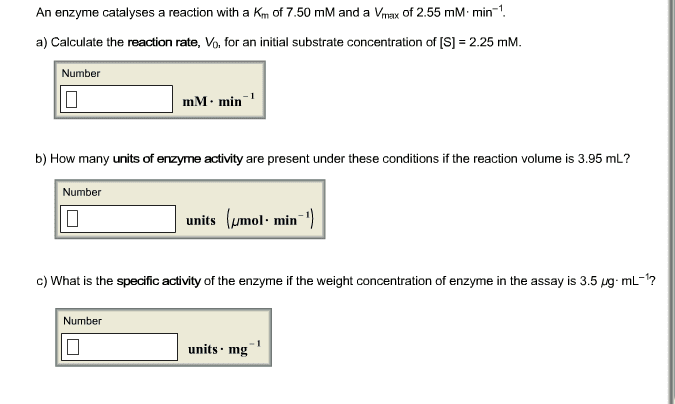1
0
watching
139
views11 Nov 2019

#An enzyme catalyses a reaction with a K_m of 7.50 mM and a V_max of 2.55 mM middot min^-1. a) Calculate the reaction rate, V_0, for an initial substrate concentration of [S] = 2.25 mM. Number mM middot min^-1 b) How many units of enzyme activity are present under these conditions if the reaction volume is 3.95 mL? Number units (mu mol middot min^-1) c) What is the specific activity of the enzyme if the weight concentration of enzyme in the assay is 3.5 mu g middot mL^-1? Number units middot mg^-1